Resource Title search

Text Search of NRICH resource titles

Or find resource begining with:Cakes and Buns

Age 11 to 14 Short Challenge Level:

Helen buys some cakes and some buns for her party. Can you work out how many of each she buys?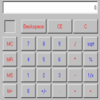Calculating the Difference: A Discussion of the Use of Calculators in the English Primary Classroom.

Age 5 to 11

Clare Green looks at the role of the calculator in the teaching and learning of primary mathematics.Calculating with Cosines

Age 14 to 18 Challenge Level:

If I tell you two sides of a right-angled triangle, you can easily work out the third. But what if the angle between the two sides is not a right angle?Calculation 2000

Age 11 to 14 Short Challenge Level:

Weekly Problem 49 - 2013
What is the value of 2000 + 1999 × 2000?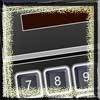Calculator Bingo

Age 7 to 11 Challenge Level:

A game to be played against the computer, or in groups. Pick a 7-digit number. A random digit is generated. What must you subract to remove the digit from your number? the first to zero wins.Calculus Analogies

Age 16 to 18 Challenge Level:

Consider these analogies for helping to understand key concepts in calculus.Calculus Countdown

Age 16 to 18 Challenge Level:

Can you hit the target functions using a set of input functions and a little calculus and algebra?Calcunos

Age 7 to 11 Challenge Level:

If we had 16 light bars which digital numbers could we make? How will you know you've found them all?Calendar Activities

Age 5 to 7

Don't get rid of your old calendars! You can get a lot more mathematical mileage out of them before they are thrown away. These activities, using cut up dates from the calendar, provide numbers to. . . .Calendar Calculations

Age 7 to 11 Challenge Level:

Try adding together the dates of all the days in one week. Now multiply the first date by 7 and add 21. Can you explain what happens?Calendar Capers

Age 11 to 14 Challenge Level:

Choose any three by three square of dates on a calendar page...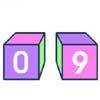Calendar Cubes

Age 7 to 11 Challenge Level:

Make a pair of cubes that can be moved to show all the days of the month from the 1st to the 31st.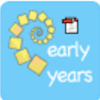Calendar Muddle

Age 3 to 5

The pictures on the timetable have been muddled up! Can you help put them back in order?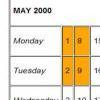Calendar Patterns

Age 7 to 11 Challenge Level:

In this section from a calendar, put a square box around the 1st, 2nd, 8th and 9th. Add all the pairs of numbers. What do you notice about the answers?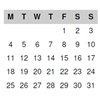Calendar Sorting

Age 7 to 11 Challenge Level:

The pages of my calendar have got mixed up. Can you sort them out?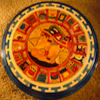Calendars

Age 5 to 14

Calendars were one of the earliest calculating devices developed by civilizations. Find out about the Mayan calendar in this article.Cambridge Maths Circle

Age 5 to 18 Challenge Level:

Problems used at the Cambridge Maths Circle.Cambridge Primary Maths Mapping Stage 1

Age 5 to 7

Cambridge Primary Maths Stage 1 activities.Cambridge Primary Maths Mapping Stage 2

Age 5 to 7

Cambridge Primary Maths Stage 2 activities.Cambridge Primary Maths Mapping Stage 3

Age 7 to 11

Cambridge Primary Maths Stage 3 activities.Cambridge Primary Maths Mapping Stage 4

Age 7 to 11

Cambridge Primary Maths Stage 4 activities.Cambridge Primary Maths Mapping Stage 5

Age 7 to 11

Cambridge Primary Maths Stage 5 activities.Cambridge Primary Maths Mapping Stage 6

Age 7 to 11

Cambridge Primary Maths Stage 6 activities.Cambridge Science Festival 2014

Age 11 to 16

Resources from Charlie's Science Festival sessions, March 2014Cambridge Science Festival 2015

Resources from Charlie's Science Festival session, March 2015Cambridge Science Festival 2018

Age 11 to 16

Resources from Charlie's Science Festival session, March 2018Can it Be

Age 16 to 18 Challenge Level:Can They Be Equal?

Age 11 to 14 Challenge Level:

Can you find rectangles where the value of the area is the same as the value of the perimeter?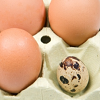Can They Be Equal? Poster

Age 11 to 14 Challenge Level:

Can They Be EqualCan You Do it Too?

Age 5 to 7 Challenge Level:

Try some throwing activities and see whether you can throw something as far as the Olympic hammer or discus throwers.Can You Find ... Asymptote Edition

Age 16 to 18 Challenge Level:

Given a sketch of a curve with asymptotes, can you find an appropriate function?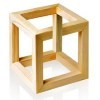Can You Find ... Cubic Edition

Age 16 to 18 Challenge Level:

Can you find cubic functions which satisfy each condition?Can You Find ... Random Variable Edition

Age 16 to 18 Challenge Level:

Can you create random variables satisfying certain conditions?Can You Find a Perfect Number?

Age 7 to 14Can You Find... Asymptote Edition

Age 16 to 18 Challenge Level:

Can you work out the equation of the function from its graph?Can You Find... Cubic Curves

Age 16 to 18 Challenge Level:

Can you find equations for cubic curves that have specific features?Can You Find... Trigonometric Edition Part 2

Age 16 to 18 Challenge Level:

Can you find trig graphs to satisfy a variety of conditions?Can You Find... Trigonometry Edition

Age 16 to 18 Challenge Level:

What graphs can you make by transforming sine, cosine and tangent graphs?Can You Make 100?

Age 11 to 14 Challenge Level:

How many ways can you find to put in operation signs (+ - x ÷) to make 100?Can You Prove It?

Age 16 to 18 Challenge Level:

Here are a collection of statements to prove, to help you to practise writing out clear mathematical proofs.Can You Traverse It?

Age 14 to 18 Challenge Level:

How can you decide if a graph is traversable?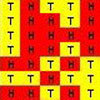Can't Find a Coin?

Age 11 to 14 Challenge Level:

Can you generate a set of random results? Can you fool the random simulator?Cancelling Fractions

Age 14 to 16 Short Challenge Level:

Can you find a fraction with the following properties?Candles

Age 14 to 16 Short Challenge Level:

What is the ratio of the lengths of the candles?Candy Floss

Age 14 to 16 Short Challenge Level:

What length of candy floss can Rita spin from her cylinder of sugar?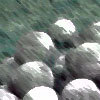Cannon Balls

Age 16 to 18 Short Challenge Level:

How high will a ball taking a million seconds to fall travel?Canny Fraction

Age 14 to 16 Short Challenge Level:

Weekly Problem 4 - 2012
What fraction of the volume of this can is filled with lemonade?Capture and Recapture

Age 11 to 16 Challenge Level:

How do scientists or mathematicians estimate the size of a population of wild animals?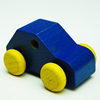Car Journey

Age 7 to 11 Challenge Level:

This practical activity involves measuring length/distance.Carbon Footprints

Age 14 to 16 Challenge Level:

Is it really greener to go on the bus, or to buy local?